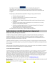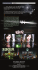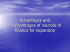CMSC 161 Exam 2

Transcription

CMSC 161 Exam 2
DEPARTMENT OF PHYSICAL SCIENCES AND MATHEMATICS
MATHEMATICS AND COMPUTING SCIENCES UNIT
CMSC 161 Special Exam
I. DEFINITION. Define the following terms briefly and concisely in the context of Computer Graphics (2 points each).
1.
2.
3.
4.
5.
6.
7.
8.
9.
10.
11.
Rendering
Aspect Ratio
Scan-conversion
Unweighted Area Sampling
Particle Systems
Homogeneous Coordinates
Affine Transformation
Vanishing Point
Frustrum / Viewing Frustrum
Depth Cueing
12.
13.
14.
15.
16.
17.
18.
19.
20.
21.
Illumination model
Mach Band effect
Ray Casting
Bounding volumes
Persistence of Vision
Color gamut
Inverse Dynamics
Pose-to-Pose
Coherence
II. PROBLEM SOLVING. Solve the following problems accordingly. Show complete and step-by-step solutions.
1.
Use Cohen-Sutherland line clipping algorithm to clip the line segment having endpoints (-5, 6) and (6, -3) on the region
bounded by the lines x=-1, y=-2, x=3 and y=3. (9 points)
2.
Give a sequence of transformation matrices in homogeneous coordinates that transforms the drawn object on the left into
the dashed object on the right. Apply the net transformation matrix to the three topmost vertices to verify your result (6
points).
3.
Given that the u-vector = (1, 0, 0), the v-vector = (0, 1, 0) and the n-vector = (0, 0, 1), what is the perspective projection of a
point (1, 2, 3) on the plane n = -3 if the eye position is (0, 0, 9) (5 points)?
Draw the effect (final position) of applying this sequence of transforms to the house (5 points).
4.
III. ESSAY. Based on what is asked on each item, answer the following questions briefly and concisely.
1.
2.
3.
4.
5.
6.
Differentiate Computer Graphics from Image Processing (3 points).
Discuss the operation of the standard Cathode Ray Tube (3 points).
Differentiate Random Scan Display from Raster Scan Display (2 points).
Briefly discuss two methods for drawing thick primitives; give the advantages of one over the other (4 points).
Differentiate the Flood Fill method from the Boundary Fill method (2 points).
Differentiate an Axonometric projection from an Oblique projection. Give advantages and disadvantages to using each (5
points).
7. Which of the visible surface algorithms discussed do you think is the easiest to implement? The hardest? Why? (4 points)
Exclude in the disadvantages the problems encountered for interpolated shading) (5 points)
9. Briefly discuss the steps in 3D viewing (4 points).
10. Briefly discuss the steps in traditional animation (3 points).
IV. BONUS.
1.
2.
How could you apply the topics discussed under Color Perception in the design of your applications and user interfaces? Explain
the importance of doing so. (4 points)
The following numbers all follow a certain rule. What is the missing number? Explain your answer (4 points).
1 2 3 1 1 _ 1 2 1 3
GOOD LUCK and GOD BLESS!

2015 LiON`S LAIR Business Plan Format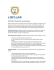FTP 122 Take Home Assignment #1 Deadline: 25.03.2015 1. Define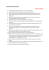Student Regd. No DMTH201:: BASIC MATHEMATICS-I 1. This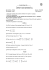Document 1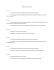Checkerboard Templates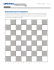Document 6540417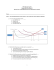PDSA Worksheet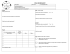Wartegg Test Form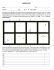Mercator Projection Winkel Tripel Projection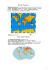Dear Applicant: Welcome to prior to completing the application for